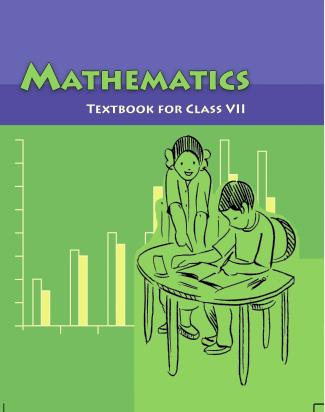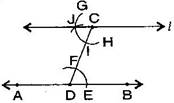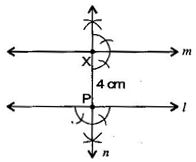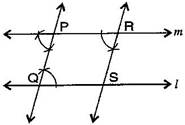1. /
2. CBSE
3. /
4. Class 07
5. /
6. Mathematics
7. /
8. NCERT Solutions for Class...

# NCERT Solutions for Class 7 Maths Exercise 10.1### myCBSEguide App

Download the app to get CBSE Sample Papers 2023-24, NCERT Solutions (Revised), Most Important Questions, Previous Year Question Bank, Mock Tests, and Detailed Notes.

NCERT solutions for Maths Practical Geometry## NCERT Solutions for Class 7 Maths Practical Geometry

###### Question 1.Draw a line, say AB, take a point C outside it. Through C, draw a line parallel to AB using ruler and compasses only.

To construct: A line, parallel to given line by using ruler and compasses.Steps of construction:

(a) Draw a line-segment AB and take a point C outside AB.

(b) Take any point D on AB and join C to D.

(c) With D as centre and take convenient radius, draw an arc cutting AB at E and CD at F.

(d) With C as centre and same radius as in step 3, draw an arc GH cutting CD at I.

(e) With the same arc EF, draw the equal arc cutting GH at J.

(f) Join JC to draw a line {tex}l.{/tex}

This the required line AB{tex}\parallel l.{/tex}

NCERT Solutions for Class 7 Maths Exercise 10.1

###### Question 2.Draw a line {tex}l.{/tex} Draw a perpendicular to {tex}l{/tex} at any point on {tex}l.{/tex} On this perpendicular choose a point X, 4 cm away from {tex}l.{/tex} Through X, draw a line {tex}m{/tex} parallel to {tex}l.{/tex}

To construct: A line parallel to given line when perpendicular line is also given.Steps of construction:

(a) Draw a line {tex}l{/tex} and take a point P on it.

(b) At point P, draw a perpendicular line {tex}n.{/tex}

(c) Take PX = 4 cm on line {tex}n.{/tex}

(d) At point X, again draw a perpendicular line {tex}m.{/tex}

It is the required construction.

NCERT Solutions for Class 7 Maths Exercise 10.1

###### Question 3.Let {tex}l{/tex} be a line and P be a point not on {tex}l.{/tex} Through P, draw a line {tex}m{/tex} parallel to {tex}l.{/tex} Now join P to any point Q on {tex}l.{/tex} Choose any other point R on {tex}m.{/tex} Through R, draw a line parallel to PQ. Let this meet {tex}l{/tex} at S. What shape do the two sets of parallel lines enclose?

To construct: A pair of parallel lines intersecting other part of parallel lines.Steps of construction:

(a) Draw a line {tex}l{/tex} and take a point P outside of {tex}l{/tex}.

(b) Take point Q on line {tex}l{/tex} and join PQ.

(c) Make equal angle at point P such that {tex}\angle {/tex}Q = {tex}\angle {/tex}P.

(d) Extend line at P to get line {tex}m.{/tex}

(e) Similarly, take a point R online {tex}m,{/tex} at point R, draw angles such that {tex}\angle {/tex}P = {tex}\angle {/tex}R.

(f) Extended line at R which intersects at S online {tex}l.{/tex} Draw line RS.

Thus, we get parallelogram PQRS.

## NCERT Solutions for Class 7 Maths Exercise 10.1

NCERT Solutions Class 7 Maths PDF (Download) Free from myCBSEguide app and myCBSEguide website. Ncert solution class 7 Maths includes text book solutions from Class 7 Maths Book . NCERT Solutions for CBSE Class 7 Maths have total 15 chapters. 7 Maths NCERT Solutions in PDF for free Download on our website. Ncert Maths class 7 solutions PDF and Maths ncert class 7 PDF solutions with latest modifications and as per the latest CBSE syllabus are only available in myCBSEguide.

## CBSE app for Students

To download NCERT Solutions for Class 7 Maths, Social Science Computer Science, Home Science, Hindi English, Maths Science do check myCBSEguide app or website. myCBSEguide provides sample papers with solution, test papers for chapter-wise practice, NCERT solutions, NCERT Exemplar solutions, quick revision notes for ready reference, CBSE guess papers and CBSE important question papers. Sample Paper all are made available through the best app for CBSE students and myCBSEguide website.Test Generator

Create question paper PDF and online tests with your own name & logo in minutes.myCBSEguide

Question Bank, Mock Tests, Exam Papers, NCERT Solutions, Sample Papers, Notes

### 11 thoughts on “NCERT Solutions for Class 7 Maths Exercise 10.1”

1. It’s very good

2. Hi Are You

3. it’s good??

4. It is very good

5. Very Useful

6. It ‘s good ??

7. Very much

8. This is very helpful and good .

9. Q.2 I think so the steps are wrong.

10. No they are correct.

11. Ok.